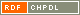# Show document

Title: Classification of edge-transitive rose window graphs Kovács, István (Author)Kutnar, Klavdija (Author)Marušič, Dragan (Author)http://dx.doi.org/10.1002/jgt.20475 English Not categorized 1.01 - Original Scientific Article IAM - Andrej Marušič Institute Given natural numbers ▫$n \ge 3$▫ and ▫$1 \le a$▫, ▫$r \le n-1$▫, the rose window graph ▫$R_n(a,r)$▫ is a quartic graph with vertex set ▫$\{x_i \vert i \in {\mathbb Z}_n\} \cup \{y_i \vert i \in {\mathbb Z}_n\}$▫ and edge set ▫$\{\{x_i, x_{i+1}\} \vert i \in {\mathbb Z}_n\} \cup \{\{y_i, y_{i+r}\} \vert i \in {\mathbb Z}_n\} \cup \{\{x_i, y_i\} \vert i \in {\mathbb Z}_n\} \cup \{\{x_{i+a}, y_i\} \vert i \in {\mathbb Z}_n\}$▫. In this article a complete classification of edge-transitive rose window graphs is given, thus solving one of three open problems about these graphs posed by Steve Wilson in 2001. group, graph, rose window, vertex-transitive, edge-transitive, arc-transitive 2010 str. 216-231 Vol. 65, no. 3 0364-9024 519.17 10241890121374 56Document is not linked to any category.

Average score: (0 votes) Voting is allowed only to logged in users.Hover the mouse pointer over a document title to show the abstract or click on the title to get all document metadata.